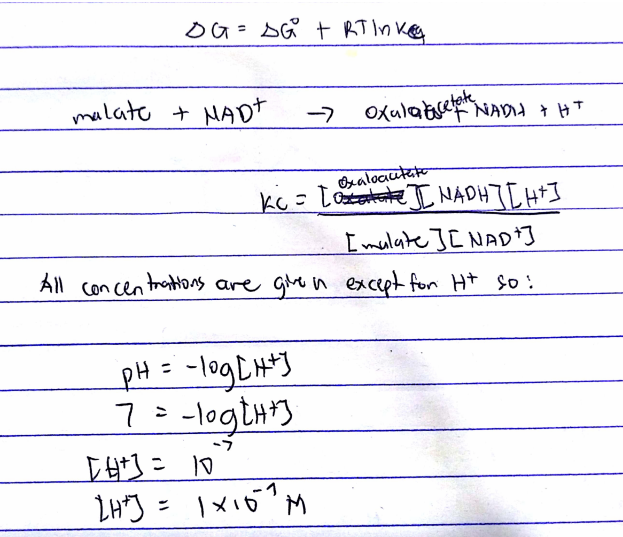# Problem: Consider the malate dehydrogenase reaction from the citric acid cycle. Given the following concentrations, calculate the free energy change for this reaction at 37.0 °C ( 310 K).  △G° for the reaction is +29.7 kJ/mol. Assume that the reaction occurs at pH 7. [malate] = 1.17 mM[oxaloacetate] = 0.210 mM[NAD+] = 480 mM[NADH] = 190mM

###### FREE Expert Solution###### Problem Details

Consider the malate dehydrogenase reaction from the citric acid cycle. Given the following concentrations, calculate the free energy change for this reaction at 37.0 °C ( 310 K).  △G° for the reaction is +29.7 kJ/mol. Assume that the reaction occurs at pH 7.

[malate] = 1.17 mM

[oxaloacetate] = 0.210 mM

What scientific concept do you need to know in order to solve this problem?

Our tutors have indicated that to solve this problem you will need to apply the Gibbs Free Energy concept. You can view video lessons to learn Gibbs Free Energy. Or if you need more Gibbs Free Energy practice, you can also practice Gibbs Free Energy practice problems.

What is the difficulty of this problem?

Our tutors rated the difficulty ofConsider the malate dehydrogenase reaction from the citric a...as high difficulty.

How long does this problem take to solve?

Our expert Chemistry tutor, Dasha took 11 minutes and 44 seconds to solve this problem. You can follow their steps in the video explanation above.

What professor is this problem relevant for?

Based on our data, we think this problem is relevant for Professor Cruz's class at USF.